# Everything You Need to Know About Tensors

TensorFlow is the go-to library for most machine learning model developers. It comes with the ease of providing standard Keras API to allow users to build their own neural networks and is equally prevalent in research and commercial applications.Source

• Tensors and NumPy arrays
• Immutability of a Tensor
• Tensors and Variables
• Operations
• Illustrations with python code

A tensor is a multi-dimensional array of elements with a single data type. It has two key properties – shape and the data type such as float, integer, or string.

TensorFlow includes eager execution where code is examined step by step making it easier to debug.

Tensor is generalized as an N-dimensional matrix.It is difficult to visualize the tensors with increasing axes, an example of Rank-3 and Rank-4 tensors is shown below:# Tensors and NumPy

The key difference between tensors and NumPy arrays is that tensors have accelerator support like GPU and TPU and are immutable.

While TensorFlow operations automatically convert NumPy arrays to Tensors and vice versa, you can explicitly convert the tensor object into the NumPy array like this: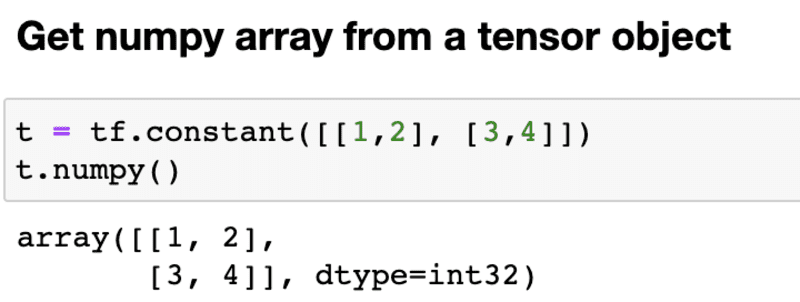# Tensors and Immutability

A tensor can be assigned value only once and cannot be updated. The tensors, like python numbers and strings, are immutable and can only be created new. In case your objective is to update the value of the tensor then you need to use variables.

Let’s learn how tensors work to understand the immutability concept. Consider a mathematical calculation like 1+1 = 2. Now, the tensor does not store the value as 2, it is the computation that is performed on the input tensors that gets executed and returns the value 2 to the output tensor.

If a random number operation is implemented on a tensor, then the number of times that operation is run, the tensor can hold different values. But it is the instructions i.eRandom number generation in this case that led the tensor to hold a different value with each run.

Now, let’s extend this concept and generalize it. If an operation O is performed on input tensors x and y, then the resulting tensor object will hold the value from the outcome of O(x,y). A tensor object will hold the same value if the operation O is deterministic and different values each time if O is a random formula. To summarize, the tensor objects refer to the function or operation that computes the output value, rather than the value itself.

# Purpose of Tensors and Variables

Unlike tensor objects, we can read tf.Variable by evaluating its read operation and write by running an assign operation. Tensor represents complex mathematical expressions like loss functions and gradients, while a variable can store a state like weight matrices and convolutional filters during training

Get Familiar with Tensor Objects:You can create a tensor with all elements filled with values 0 or 1 using tf.ones and tf.zeros.Note that the default data type shown above is float. A tensor can have only one data type at a time. You can specify the data type as an integer below:Alternatively, you can change the data type using tf.cast. We have created a 1-D tensor with a value of 4.5.Note that we have not specified the data type explicitly, it is float32. You can check the data type using dtype, like how you accessed the NumPy array: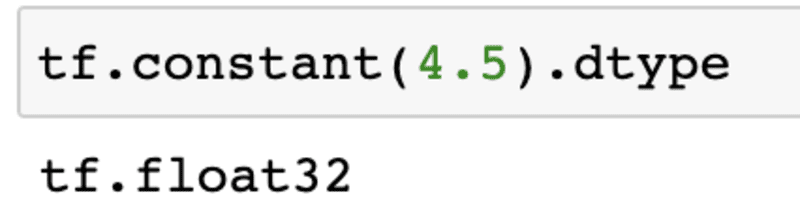The tensor with float data type has changed to integer: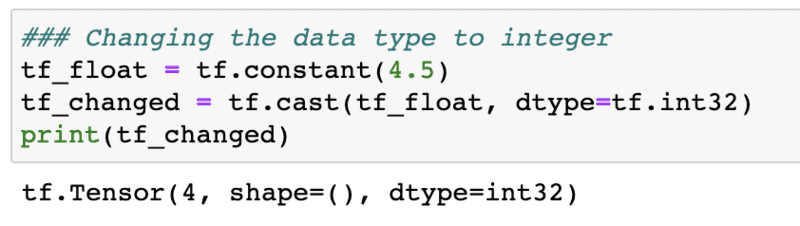Like creating a tensor with all elements filed with value 1, you can also create a tensor of a given shape with all elements set to 0: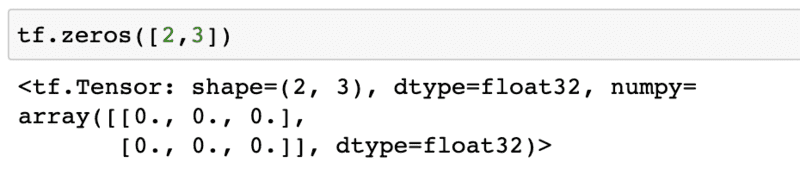# TensorFlow Variable

It stores the persistent state of the variables. The variable constructor has a Boolean parameter ‘trainable’ that distinguishes the trainable variable that stores model parameters from the non-trainable variables. This allows easy tracking of variable values and saving them to training checkpoints or SavedModels that include serialized TensorFlow graphs.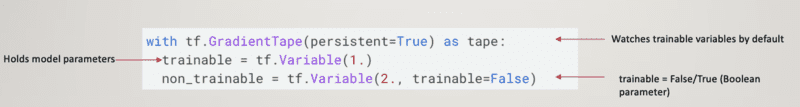tf.Variable objects are built on top of tf.Tensor objects and have properties like name, value, data type, shape, etc. If you want to be able to trace the values of updated model parameters during training, give a ‘name’ argument in the input while creating the variable. In the absence of any specific name, TensorFlow assigns a default name as shown below:Notice the name “var_example” while creating a variable:# Operations

It is a graph node that takes tensors as an input, performs computation on them, and outputs tensor objects. There is a rich list of all the operations that can be performed on Tensors.

An example to perform addition and multiplication operation on two input tensors is shown below:Similarly, there are common operations like tf.subtract(x,y), tf.multiply(x,y), tf.div(x,y), tf.pow(x,y), tf.exp(x), tf.sqrt(x) etc.

With this, we have come to an end to this post where we explained tensors through visualization, the difference between tensor objects and variables. We also covered how tensors are different from variables and when to use them. Next, we illustrated through examples various operations that can be performed on tensors.

# References

Vidhi Chugh is an award-winning AI/ML innovation leader and an AI Ethicist. She works at the intersection of data science, product, and research to deliver business value and insights. She is an advocate for data-centric science and a leading expert in data governance with a vision to build trustworthy AI solutions.